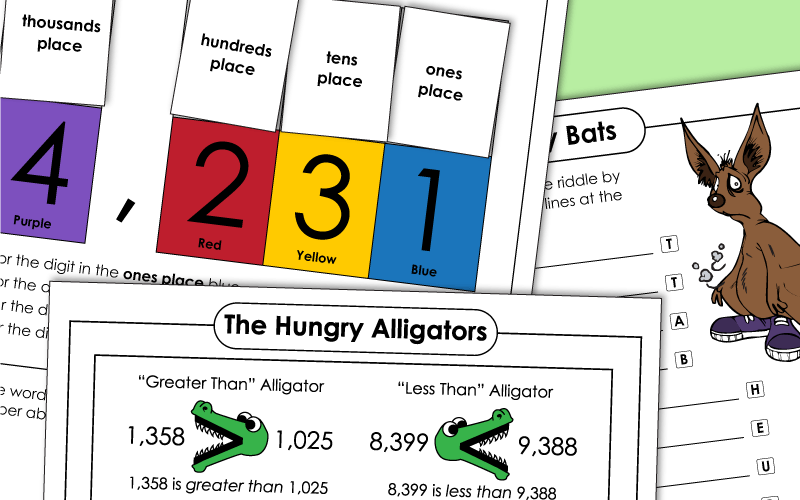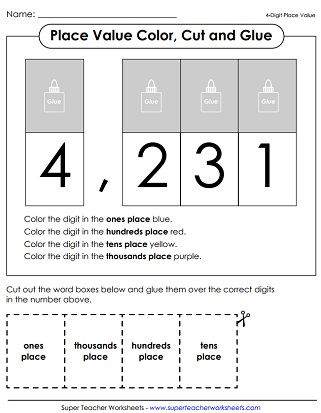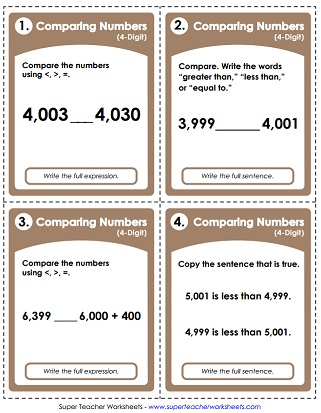# Place Value: 4 Digits (Thousands)

Below you'll find many printable worksheets and lessons for reviewing 4-digit place value with your students. Practice writing numbers in expanded notation, ordering from greatest to least, counting base-10 place value blocks, and more.## Place Value

Place value blocks (base 10 blocks) and expanded form.
Use scissors to cut out the place value squares (ones, tens, hundreds, thousands). Glue each above the correct digit. Then color each digit according to the directions.
Four different students are each given a card with a large digit on it. The caller reads a number, such as one thousand, five hundred seven. Students hold up their numbers for the class to see, and arrange themselves in a line to make the number.
Practice place value up to the thousands place with this printable puzzle. Match the base ten blocks with the correct number.
Tell how many thousand cubes, hundreds flats, ten sticks, and ones cubes are in each number.
What 4-digit number is represented by the thousands, hundreds, tens, and ones in the pictures of place value blocks?
Read the 4-digit numbers and tell how many thousands, hundreds, tens, and ones are in each.
The ancient Egyptian place value system is very similar to our own. In this activity, students will learn to read and write ancient Egyptian numbers using a simple place value method.
This is a continuation of the other Ancient Egyptian worksheet. This time, kids use Ancient Egyptian numbers as a model for creating their own base-10 number system.
Cut the rectangles and match the equivalent numbers. Then tell whether each is written in standard form, word name form, or expanded form.
Write the four-digit numbers that are shown on the abacus illustrations.
Use place value blocks to build 4-digit numbers up to 9,999.
On this math worksheet, students will write the four-digit numbers in standard form. Then match the numbers with the letters to find the answer to a funny riddle.
Make the largest and smallest number possible by arranging the given digits.
Students must use the digits in the box to find the answers to the questions; Up to 4 digits.
Rearrange digits on the chart to build 4-digit numbers.
Students add 1, 10, and 100 to each number on the table.  They then subtract 1, 10, and 100 from each number.
Print these base-10 blocks on card stock and cut them out. Includes thousands, hundreds, tens, and ones.

## Special Number

This PDF has lots of PlaceVal questions for the number 1,507. Circle the base-ten blocks that show this number. Tell whether it is odd or even. Tell how many 1000s, 100s, 10s, and 1s are in this number.
This printable activity has lots of p.v. questions to go along with the number 2,354. Kids will circle the P.V. cubs that represent this number, write the number in expanded form, and complete the number line.
The special number on this document is four thousand, ninety-two. Students answer a set of place value questions to go along with this number.
The feature number is six thousand, eight hundred twenty. Kids must compare this number to other numbers using <, >, and =. Also, write the value of the digits, write the number in expanded form, and finish the number line.
This S.N. worksheet features the number three thousand, one hundred twenty-six. Students must use place value and arithmetic skills to answer the questions.

## Ordering Numbers

Cut the sheets of cards apart.  Students sort them from least to greatest.
On this worksheet, students order numbers, from smallest up to the largest.
These dancing pigs have 4-digit numbers on them.  Sort the pigs in order from least to greatest.

## Comparing Numbers

This is a two-player place value game. Players roll a six or ten-sided die four times to make numbers. They compare the numbers using the <, >, or = symbol.
Students must figure out which number is greater. Cut out the alligator pictures and paste them into the correct spaces on the worksheet.
"Greater Alligator" and "Less Alligator" are gobbling up numbers with large values.  Students attach the correct alligator symbol to each problem. Then they write out the correct answer in words.
These task cards can be used for instruction with a document camera. Or use them for classroom scavenger hunts, math games, learning centers, or small group instruction.
Practice comparing numbers.  In part 1, students use <, >, and = symbols. In the second part, students draw a ring around the greater number. In the third part of this worksheet, students determine the smaller number. On the final part, kids will write the out the words greater than or less than.
Compare four-digit numbers using words and math symbols. (example: 3,343 < 3,353)

Students read the 4-digit numbers aloud and the teacher scores them.
Say the names of the 4-digit numbers.  Includes a homework page and a quiz.
Spin the wheel and try to read each 3 and 4-digit number aloud. Students can read numbers to a classmate, a parent, or a teacher.
5-Digit Place Value

Use these worksheets and activities if you're teaching students how to read and write 5-digit numbers.

## Sample Worksheet ImagesMy Account
Site Information